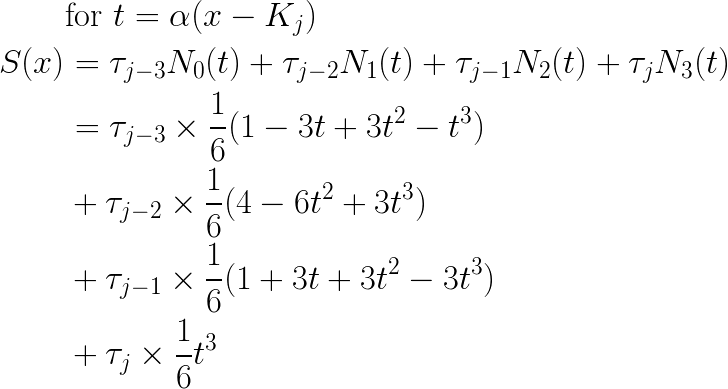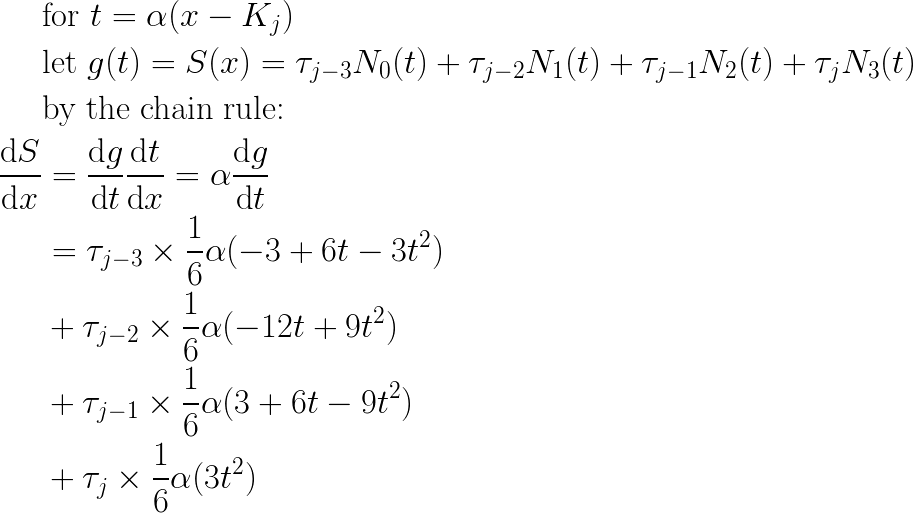In my previous post I derived the standard-form polynomial coefficients for cubic B-splines. As part of the same project, I also need to add a feature that allows the library user to declare equality constraints of the form (x,y), where S(x) = y. Under the hood, I am invoking a convex optimization library, and so I need to convert these user inputs to a linear equation form that is consumable by the optimizer.

I expected this to be tricky, but it turns out I did most of the work already. I can take one of my previously-derived expressions for S(x) and put it into a form that gives me coefficients for the four contributing knot points Kj-3 ... Kj:Recall that by the convention from my previous post, Kj is the largest knot point that is <= x.

My linear constraint equation is with respect to the vector I am solving for, in particular vector (τ), and so the equation above yields the following:In this form, it is easy to add into a convex optimization problem as a linear equality constraint.

Gradient constraints are another common equality constraint in convex optimization, and so I can apply very similar logic to get coefficient values corresponding to the gradient of S:And so my linear equality constraint with respect to (τ) in this case is:And that gives me the tools I need to let my users supply additional equality constraints as simple (x,y) pairs, and translate them into a form that can be consumed by convex optimization routines. Happy Computing!